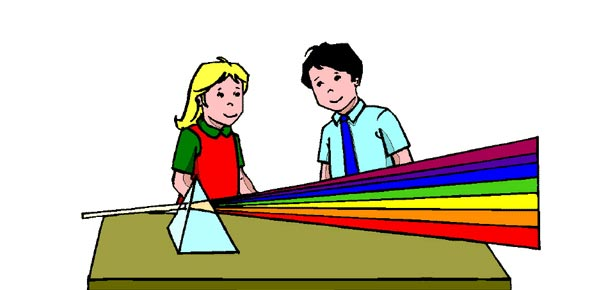# 9 Light And Sound

15 Questions | Total Attempts: 107SettingsQuiz on Light and Sound content covered.

• 1.
Sound needs ___________ to vibrate to transmit the wave.
• A.

Particles

• B.

Energy

• C.

Gas

• D.

Liquid

• 2.
Sound travels fastest in air because the particles are spread furthest apart.
• A.

True

• B.

False

• 3.
In a sound wave, the places where the particles are furthest apart are called the:
• A.

Compresssions

• B.

Rarefactions

• C.

Wavelength

• D.

Amplitude

• 4.
What is the correct relationship for the pitch and frequency of sound?
• A.

The higher the pitch, the higher the frequency.

• B.

The lower the pitch, the higher the frequency.

• C.

The higher the pitch, the lower the frequency.

• D.

There is no relationship between pitch and frequency.

• 5.
What is the correct relationship between the amplitude of sound and the volume?
• A.

The louder the sound, the smaller the amplitude.

• B.

The louder the sound, the higher the amplitude.

• C.

The quieter the sound, the higher the amplitude.

• D.

There is no relationship between volume and amplitude of sound.

• 6.
For transverse and longitudinal waves, the higher the frequency...
• A.

The longer the wavelength

• B.

The shorter the wavelength

• C.

The wavelength remains the same

• 7.
Many rays of light make up a beam.
• A.

True

• B.

False

• 8.
The Law of Reflection states that the angle of incidence is equal to the angle of reflection.
• A.

True

• B.

False

• 9.
Ray boxes in Science are used to _________  the path of light.
• 10.
A concave mirror causes the light to diverge.
• A.

True

• B.

False

• 11.
Refraction of light occurs because the _______ of light changes as it goes from one medium to another.
• A.

Angle

• B.

Speed

• C.

Direction

• D.

Frequency

• 12.
A diverging lens is one that has a ____________ shape.
• A.

Biconcave

• B.

Biconvex

• C.

Flat

• D.

Irregular

• 13.
The clear part of the eye that is attached to the sclera is called the...
• A.

Lens

• B.

Cornea

• C.

Retina

• D.

Pupil

• 14.
Which technology relies on mirrors or lenses for an image to be produced?
• A.

Telescope

• B.

Microscope

• C.

Camera

• D.

All of them do

Related TopicsBack to top